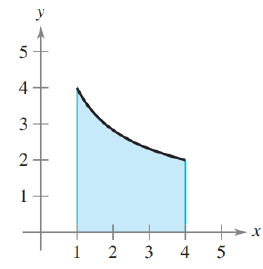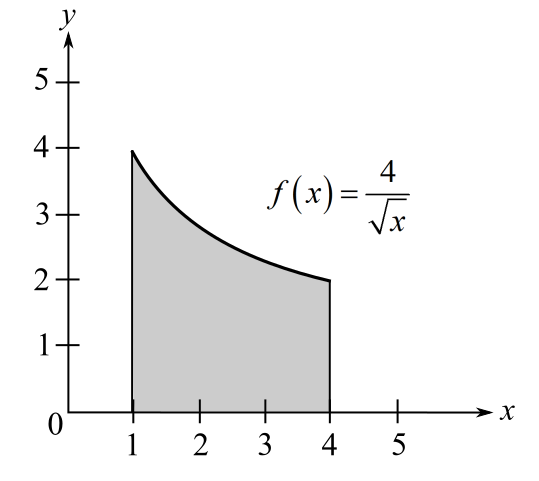Chapter 5, Problem 55RE### Calculus: An Applied Approach (Min...

10th Edition
Ron Larson
ISBN: 9781305860919

#### Solutions

Chapter
Section### Calculus: An Applied Approach (Min...

10th Edition
Ron Larson
ISBN: 9781305860919
Textbook Problem
1 views

# Finding Area by the Fundamental Theorem In Exercises 53-58, find the area of the region. f ( x ) = 4 xTo determine

To calculate: The area of region bounded by graph of function f(x)=4xExplanation

Given Information:

The provided graph of function f(x)=4x.

Formula used:

Area of function f(x) bound from x=a and x=b is given by

Area=abf(x)dx

The fundamental theorem of calculus:

abf(x)dx=F(b)F(a)

Here, F is function such that F(x)=f(x) for all x in [a,b].

Calculation:

The provided graph of function f(x)=4x.

The graph of function f(x)=4x is parabola bounded from x=1 and x=4

### Still sussing out bartleby?

Check out a sample textbook solution.

See a sample solution

#### The Solution to Your Study Problems

Bartleby provides explanations to thousands of textbook problems written by our experts, many with advanced degrees!

Get Started

#### In Exercises 2340, find the indicated limit. 40. limx3xx2+72x2x+3

Applied Calculus for the Managerial, Life, and Social Sciences: A Brief Approach

#### In problems 59 and 60, simplify each expression. 60.

Mathematical Applications for the Management, Life, and Social Sciences

#### True or False: limx6x3+8x29x+75x34x+30=limx6x35x3.

Study Guide for Stewart's Single Variable Calculus: Early Transcendentals, 8th